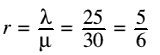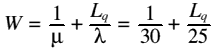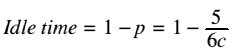# Queues in a Bank

## Introduction

As a rule people dislike queueing in banks, and so in order to improve the level of service a bank manager is interested to find out:
1. The average number of people waiting in the bank (i.e. the queue length)
2. How much of the cashiers' time is spent idle (as a percentage of their time)
Depending on how many tellers she employs during lunchtime.  She is prepared to employ up to 5 cashiers, but not less than 1.

## Model Assumptions and Details

• The distribution of the length of time it takes the cashiers to carry out a task is exponential, with mean 2 minutes and standard deviation of 5/4 minutes
• There is virtually no limit on the queue length, as the bank has a large floor area
• Customers arrive in a Poisson distribution, with mean of 25 per hour
• Service is done on a first come first serve basis
• This is an M/M/c queue, where 1 =< c =< 5

## Symbols

 Symbol Meaning, and value if in assumptions c Number of cashiers (servers) Lq Average length of the queue W Percentage of cashiers idle (waiting) timeMean arrival rate (25 per hour)1/(mean time to server customers) = 30 per hour p Average amount of work for each server, per hour

## Calculations

From the above we can easily calculate the following values, which are used to work out Lq and W:p0 and Lq, W and the idle time can be calculated using the following equations:The results of these equations for 1 to 5 cashiers are summarised in the following table:
 Cashiers p r^n / n! p0 Lq W Idle 1 0.833 0.833 0.167 4.167 0.2 0.167 2 0.417 0.347 0.412 0.175 0.04 0.583 3 0.278 0.096 0.432 0.022 0.034 0.722 4 0.208 0.02 0.434 0.003 0.033 0.792 5 0.167 0.003 0.435 0.000 0.033 0.833
From the table it can be seen that either 1 or 2 cashiers should be employed, as this will keep a short queue, but without the cashiers being idle too much of the time.

Henry Morgan, 14 June 1999